Type I and Type II Errors

The Type I error is the error of rejecting the null hypothesis when the null hypothesis is correct, and the Type II error is the error of not rejecting the null hypothesis when the null hypothesis is incorrect. The level of significanceis the probability of making a Type I error. The Type II error depends on the hypothetical reference of the alternative hypothesis, and the Type II error probabilityis defined as the probability of not rejecting the null hypothesis when a specific alternative reference is true. The power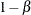is then defined as the probability of rejecting the null hypothesis at the alternative reference.

In a sequential design, if the maximum information and alternative reference are not both specified, the critical values are created such that both the specified Type I and the specified Type II error probability levels are maintained in the design. Otherwise, the critical values are created such that either the specified Type I error probability or the specified Type II error probability is maintained.

One-Sided Tests

For a K-stage group sequential design with an upper alternative hypothesis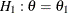and early stopping to reject or accept the null hypothesis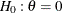, the boundaries contain the uppercritical values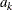and uppercritical values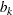,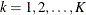. At each interim stage,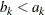, the null hypothesisis rejected if the observed statistic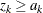,is accepted if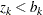, or the process is continued to the next stage if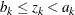. At the final stage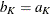, the hypothesis is either rejected or accepted.

The overall Type I error probabilityis given by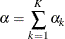where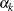is thespending at stage k. That is, at stage 1,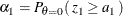At a subsequent stage k,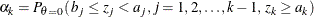Similarly, the Type II error probability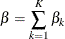where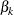is thespending at stage k. That is, at stage 1,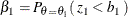At a subsequent stage k,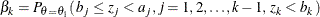With an upper alternative hypothesis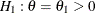, the poweris the probability of rejecting the null hypothesis for the upper alternative.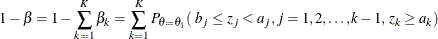For a design with early stopping to rejectonly, the interim uppercritical values are set to,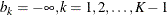, and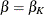. For a design with early stopping to acceptonly, the interim uppercritical values are set to,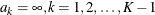, and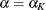.

Similarly, the Type I and Type II error probabilities for a K-stage design with a lower alternative hypothesis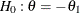can also be derived.

Two-Sided Tests

For a K-stage group sequential design with two-sided alternative hypotheses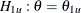and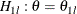, and early stopping to reject or accept the null hypothesis, the boundaries contain the uppercritical values, uppercritical values, lowercritical values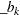, and lowercritical values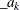,. At each interim stage,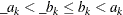, the null hypothesisis rejected if the observed statisticor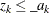,is accepted if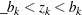, or the process is continued to the next stage ifor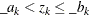. At the final stageand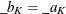, the hypothesis is either rejected or accepted.

The overall upper Type I error probability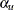is given by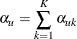where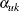is thespending at stage k for the upper alternative. That is, at stage 1,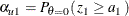At a subsequent stage k,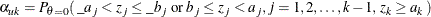Similarly, the overall lower Type I error probability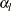can also be derived, and the overall Type I error probability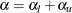.

The overall upper Type II error probability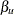is given by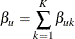where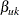is the upperspending at stage k. That is, at stage 1,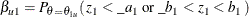At a subsequent stage k,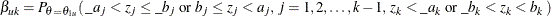With an upper alternative hypothesis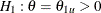, the power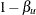is the probability of rejecting the null hypothesis for the upper alternative: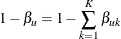which is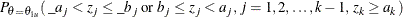The overall lower Type II error probability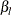and power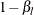can be similarly derived.

For a design with early stopping only to reject, both the interim lower and uppercritical values are set to missing,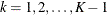, and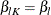,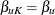. For a design with early stopping only to accept, the interim uppercritical values are set to,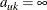, and the interim lowercritical values are set to,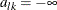,, and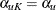,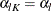.